Tachometer FAQIs it possible to measure with reflective marks (HT-011) cut into smaller pieces?
(Applicable models: HT-4100/5100/5200/5500, LG-916/930, FG-1200, and PP-940)It is possible although measurement ranges (both of the detection distance and the rotational speed) change. The measurement ranges depend on the conditions as follows.

An influence of measurement distance

Let us suppose that we cut a piece of reflective mark into half size, for a simple example. If you cut a piece of reflective mark into half size, an amount of reflected light also will be half, and it means that amount of received light by the tachometer will decrease. Therefore, you have to shorten the measurement distance to obtain the same amount of light from a reflective mark of the provided size (12mm × 12mm). If the area of the provided reflective mark is expressed by S, and the maximum detection distance when using the provided reflective mark is expressed by L max; as you can see from the following figure, the lower expressions are led.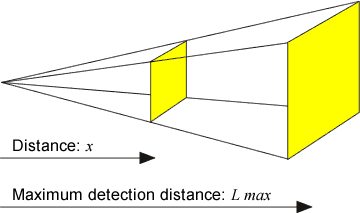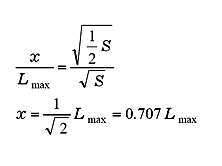Thus x (the maximum detection distance when using the half-size reflective mark) will be approx. 70% of the maximum detection distance when using the provided reflective mark.

An influence of rotational speed

When you use a smaller reflective mark, reflecting time will be shorter than that of the larger one, although the rotating speed is the same. If the reflecting time is shorter than a certain time (minimum reflecting time for the light reception), the amplifier of the tachometer does not respond.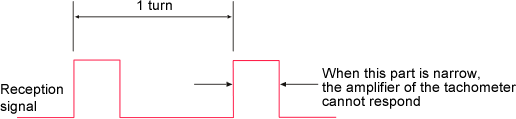As you can see from the following figure, if the rotational speed is expressed by n(r/min), and the distance from the rotation center to the reflective mark is expressed by r, the reflecting time Δt of the reflective mark with a width of Δd is as follows.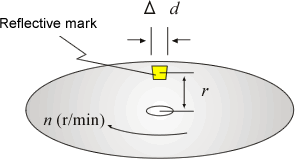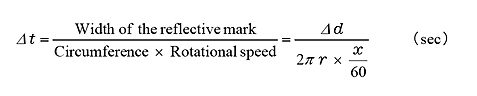The minimum time of Δt = Δt min (minimum reflecting time for the light reception) depends on the models, and the approximate values of each model are shown in the following table.

Model

Minimum reflecting time Δt min( ms )

HT-4100 0.2
HT-5100/5200 0.2
LG-916 0.6
LG-930 0.5
FG-1200 0.1
PP-940 0.05

Maximum rotational speed for the detection that is expressed by the minimum reflecting time for the light reception Δt min is as follows.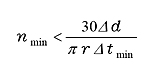In addition, since the tachometer also requires a period that is not receiving the reflecting light (the part without the reflective mark) at least as the same length as the period that is receiving it, the maximum rotational speed is expressed by the expression as follows.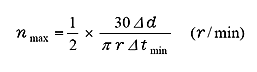Therefore, if the width of the reflective mark (Δd) is half of the provided one, the maximum rotational speed (n max) is also half. This is only by a calculation; actually, the rotational speed should be a little slower than the result of the calculation.

To hasten the maximum rotational speed for the detection

Now you know, to hasten the maximum rotational speed, you should make Δd large in the upper expression. In other words, if you affix 2 or 3 pieces of reflective mark closely, the maximum rotational speed will be twice or 3 times.

Revised:2017/04/28